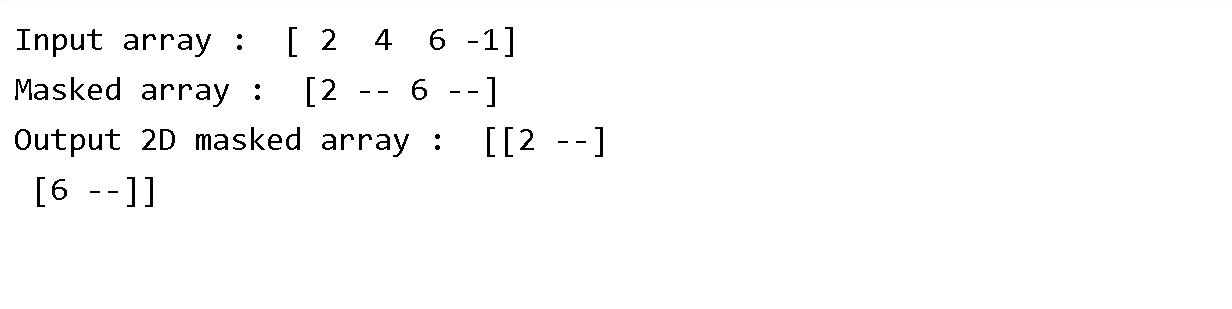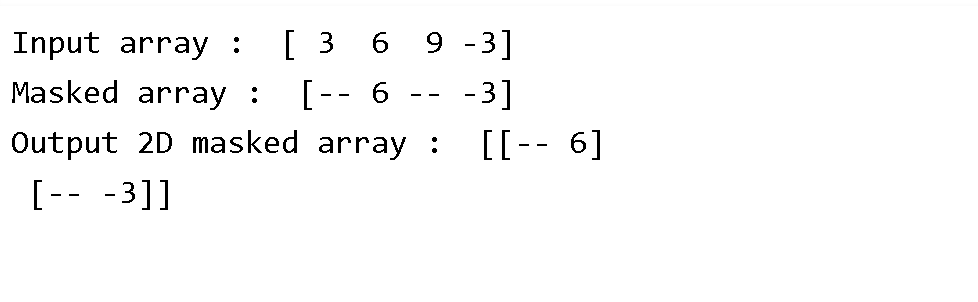### Python Numpy Masked Array reshape()

Description
NumPy generally stands for Numerical Python and it is a general purpose array processing package.it always provides a high performance multidimensional array object. Numpy is usually for creating an array of n dimensions.

Method Name :numpy.ma.reshape(shape, order)
Description : This function is used to give a new shape to the masked array without changing its original data. It returns an array with a new shape but containing its data, which results in the view
on the original array, if this is not possible a VALUE ERROR is raised.

Parameter Name: shape
Description : int or tuple of ints
The new shape should be compatible with the original shape. If an integer is supplied then the result will be a 1-d array of that length.

Parameter Name: order
Description :C,F,A,K optional
By default 'C' index order is used
The element of a is read using this index order.

'C' means to index the element in c-like order, with the last axis index changing fastest, and the first axis index changing slowest .
'F' means to i8ndex the element in FORTRAN like index order, with the first axis index changing fastest and the last axis index changing slowest.
'A' means to read the element in FORTRAN like index order if m is FORTRAN contiguous in memory, c like order otherwise.
'K' means to read the elements in the order they occur in memory, except for reversing the data when strides are negative.

Return : This function returns the new view on the array.

The first step would be to give syntax
command:
numpy.ma.reshape(shape, order)

Example :
Code :
import numpy as np import numpy.ma as ma # creating input array in_arr = np.array([2, 4, 6, -1]) print ("Input array : ", in_arr) mask_arr = ma.masked_array(in_arr, mask =[0, 1, 0, 1]) print ("Masked array : ", mask_arr) out_arr = mask_arr.reshape(2,2) print ("Output 2D masked array : ", out_arr)

Output :Example :
Code :
import numpy as np import numpy.ma as ma # creating input array in_arr = np.array([3,6,9,-3]) print ("Input array : ", in_arr) mask_arr = ma.masked_array(in_arr, mask =[1,0,1,0]) print ("Masked array : ", mask_arr) out_arr = mask_arr.reshape(2,2) print ("Output 2D masked array : ", out_arr)

Output :